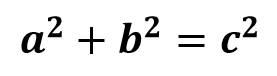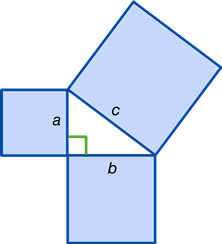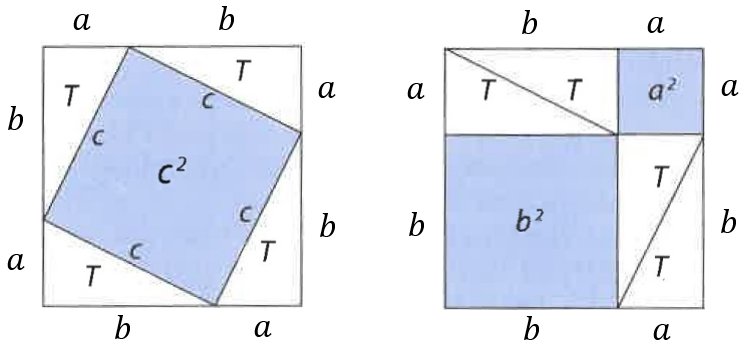Maths
The Pythagoras Puzzle
Author
Tommie Zetterlund; Bromangymnasiet Hudiksvall
Target Group
13-17 years old students
Brief Description
The Pythagorean theorem has been given numerous proofs – possibly the most for any mathematical theorem. This puzzle is a way for the students to verify the theorem themselves.

Share

# The Pythagoras Puzzle

In mathematics, the Pythagorean theorem, also known as Pythagoras's theorem, is a fundamental relation in Euclidean geometry among the three sides of a right triangle. It states that the square of the hypotenuse (the side opposite the right angle) is equal to the sum of the squares of the other two sides. The theorem can be written as an equation relating the lengths of the sides a, b and c, often called the "Pythagorean equation":where c represents the length of the hypotenuse and a and b the lengths of the triangle's other two sides.The theorem has been given numerous proofs – possibly the most for any mathematical theorem. This puzzle is a way for the students to verify the Pythagorean theorem themselves.

1. Have the student work in groups.

2. Distribute the copying material and scissors to the groups.

3. Instruct them to put the four triangles in the square to somehow form the area a2.

4. After they have managed to form the area c2 they should rearrange the triangles to try to form the areas a2 and b2.

5. Instruct them to discuss if this verifies the theorem. And encourage them to draw their own right-angled triangles to try to verify if this is true for all right angled triangles.

Teachers guide```Note 1: Before the introduction to this puzzle it is recommended to talk a little about the history of
the Pythagorean theorem and Pythagoras himself.
Note 2: The students can use a spreadsheet application to further examine if the theorem is true for
different values of a and b.```

## Related files

 The Pythagoras Puzzle_copying material_ENG 421 KB The Pythagoras Puzzle_teachers manual_ENG 424 KB
If you have tried out a lesson, please fill the next form to help us to improve it: